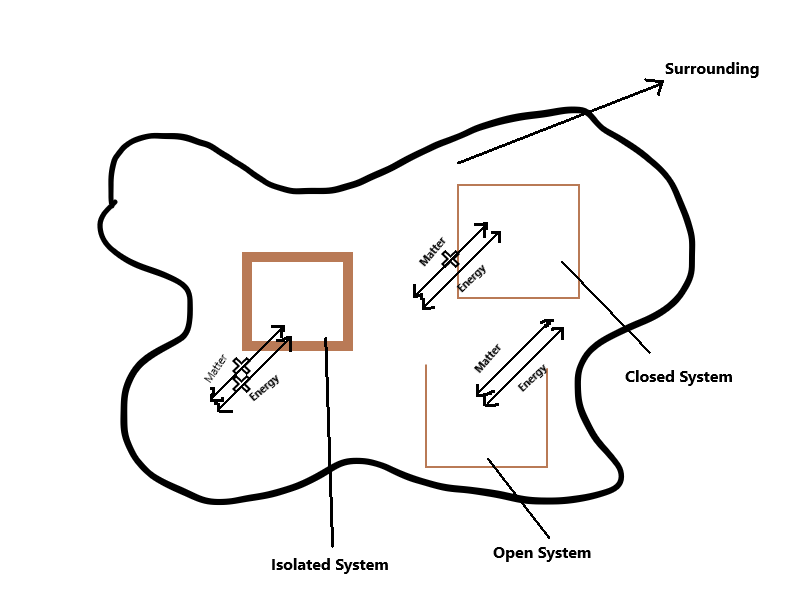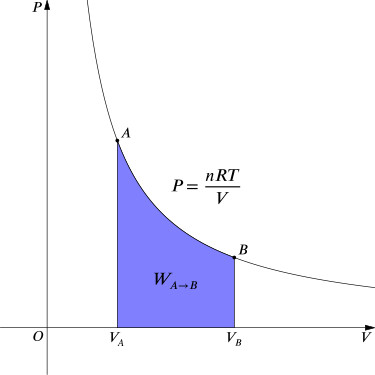# Learn First Law of Thermodynamics in Simple Language

Do you find it hard to understand first law of thermodynamics and its problems? Do not worry we have you covered ! The first law of thermodynamics states that; for any spontaneous or non spontaneous reaction the sum of energy of the system and its surrounding remain constant. In simple English the law states that the energy can never be created nor destroyed; but can only change from one form to another.

You can find example for this statement in form of electric heater, fan and i.c engine which convert one form of energy to another. To better understand the law in a step by step approach without much confusion; lets start from basics.

## Basics OF Thermodynamics

Thermodynamics is the part of science that deals with energy conversion in its different forms. It helps understand whether a particular change ( physical or chemical ) will occur under a specific condition; ignoring internal structure of its atom and molecule. There are three basic laws of thermodynamics that generalize into first , second and third law of thermodynamics.

#### System And Surrounding

The part of universe under thermodynamic consideration is known as system while everything else is called its surrounding. There is a thin physical or imaginary line in between known as boundary; which divide  the system from surrounding. For example 500 ml water in a kettle is the system while the air inside along with the kettle itself is the surrounding. The system is further classified into three types based upon the nature of its boundary:-1 ) Isolated System: The system with a closed and isolated boundary which do not allow for the transfer of matter or energy to and from the surrounding is called isolated system. For example a insulated water bottle with hot water inside; water can not escape the container nor does the heat or the water vapour.

2 ) Closed System: A system with a closed boundary which do not allow for physical transfer of matter; but allow energy to flow to and from the system and its surroundings in form of heat, radiation and work. For example water in a pressure cooker can not transfer the matter ( until depressurized ); but allow heat energy to flow from the system to outside surrounding.

3 ) Open System: A open system does not have any physical boundary and is open to the surrounding allowing both matter and energy to flow without any restriction. For example water boiling in a jar or open kettle; where the water vapour and heat energy is lost to the surrounding.

## Thermodynamic Process

When a system undergoes change in its state or any of its condition such as temperature, pressure or volume then the operation is termed as thermodynamic process. You might already know some of these thermodynamic process; but for the scale of good let’s discuss various types of thermodynamic process:-

1 ) Isothermal Process: The thermodynamic process in which the temperature remains constant during the change of state. For example evaporation of water at 100 degree centigrade; at 100 degree the water change its state from liquid to gas under constant temperature.

2 ) Adiabatic Process: The thermodynamic process in which there is no flow of heat in or out of the system.  For example the compression and expansion of air due to sound waves propagating through the medium.3 ) Isobaric Process: These are the thermodynamic process in which pressure remains constant during the change of state. For example boiling water in a open system will lead to change of state without change in pressure as the vapor expands at atmospheric pressure.

4 ) Isochoric Process: the thermodynamic process in which the volume remains constant during the change of state. For example when water is boiler in a closed container the state will change at constant volume.

5 ) Reversible Process: A thermodynamic process which go for an indefinite time with any given point in between the initial and final state is in equilibrium; so that it can be recovered with certain change in the state at any time. There is no practical example of reversible process.

6 ) Irreversible Process: The thermodynamic process that happen in one step from start to finish and can not be reversed is known as irreversible process. For irreversible process the system is at equilibrium only at start and end of the process. For example two gas when mixed together is a irreversible process.

7 ) Spontaneous Process: A thermodynamic process is defined to be spontaneous if and only if the process take place without any external influence or work. The spontaneous process must always proceed of its own unaffected by the external factors.

8 ) Non-Spontaneous Process: A non-spontaneous process is the exact opposite of the spontaneous process. It requires external influence and effort to proceed forward in form of work and catalyst in reaction.

## Internal Energy

A internal energy of a system is the total ( kinetic + potential ) energy of the random molecules under motion. In other words it is the total energy of a system which remains constant. For example air inside a balloon have some energy; now if the balloon flow with the wind at 0.5 km/hr does the internal energy of the closed system i.e balloon change? No ! Now if we catch the balloon and press it; will the internal energy change? No ! In any case there can be a change in pressure, kinetic or potential energy of the system but its internal energy remains same.

In even better way; suppose you have one brother whom you have given your 5 rupees ( consider it your currency ) and have left with 10 rupees. Now in a closed system of your family ( You + your brother ) the total money remains constant i.e 15 rupees. Internal energy is just similar to that and is represented by ” ΔU ” and is given by the sum of of heat transfer and negative work done according to the first law of thermodynamics.

#### ΔU = Q – W

Note: For a thermodynamic process from state A to B the change in internal energy is given by ΔU = U2 – U1 and is always independent of the path or the process.

## Work Done On The System For Isothermal ProcessThe image is in public domain and taken from wikipedia ( Representation of work done on isothermal process system )

Consider a closed system of cylinder with a frictionless piston moving upward. Now the ideal gas in the system is expanding reversibly from v1 to v2 while the pressure decrease from p1 to p2 at a constant temperature. Now as this is a reversible process it will go through a infinite number of steps in between v1 and v2.

∴,

As we know work done ( W ) = Force ( F ) X distance / displacement ( h )

So, for one step in the infinite series;

dw = F x dl

= P X A X dh

= p x dv ( as, volume = area x height )

As we have learn that the work done on the system is negative; the total work done on the system is:

And from ideal gas equation we know P X V = nRT

$\therefore \mathrm{W = –}\mathrm{nRT}ln\frac{{V}_{2}}{{v}_{1}}$

And From ideal gas equation we know P1V1 = P2V2

Or,

## First Law of Thermodynamics

Suppose you are in a auditorium with 200 others and there is no air conditioner. Now if the auditorium is closed it works well as an example of closed system; As you all exhale carbon dioxide along with some heat increasing the heat of the auditorium exponentially. Now you all will start to feel the heat and start to sweat.

Your body heat is absorbed and taken away by your sweat which then evaporate further increasing the auditorium temperature along with stinking smell. Now this process of increasing auditorium temperature through lost heat is a perfect example of first law of thermodynamics. Here in the closed system of auditorium no heat is lost but is only transfered from your body to atmosphere.

Let’s understand it in a different way; If you know what a pendulum is, initially when you set it in motion, it start oscillating. If you don’t know much about thermodynamics, the pendulum might seems, that it will go on forever without any external power / force / energy powering it (A perpetual motion machine, in another words); but pendulum is not a perpetual motion machine. It is so because perpetual motion is impossible according to the law of physics, and one of the reason is first law of thermodynamics.

As a Thermodynamics system work, it loses heat, when work is done on the system, it gains heat. Heat is converted into work and work is converted into heat. Together the work and heat transferred into or out of the system represent the change in its Internal energy.

It is important to know, if heat is transferred into the system, Heat Q is positive and if heat Q is transferred out of the system Q is negative and if Work W is done by the system, work W is positive, if work is being done on the system, it is negative.

Here you’ll notice that first law of thermodynamics describe only two factors, work and heat as effecting the change in internal energy. That is because as long as system is closed there just aren’t any other factor involved. So the amount of heat lost by the system is exactly equals to amount of work done by the system and visa-versa.

This stated, basically the first law of thermodynamics is a way to describe conservation of energy. There’s always some kind of heat lose through friction, even this tiny amount eventually leads to run out of energy needed to drive the work; and therefore pendulum is not perpetual motion machine, it relies on the force exerted initially by hand for oscillation.

##### Can’t Find What You Are Looking For?

Why not Request your own Topic !

### 3 thoughts on “Learn First Law of Thermodynamics in Simple Language”

1.what is the main caused by a boiler explosion?

•2.